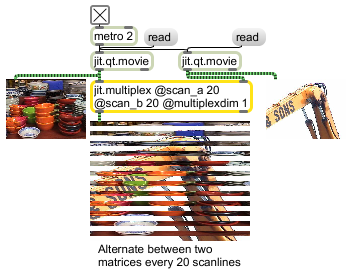# jit.multiplex

Multiplex (interleave) two matrices into one matrix

## Description

The jit.multiplex object takes two matrices as input, and derives a single output matrix from it by interleaving the input matrices across a specified dimension. Specifically, the object takes a set of scanlines across the first input matrix, placing these into the output matrix, then takes a set of scanlines across the second input matrix, appending them to the output matrix, alternating between the two input sources until their data is exhausted.

## Matrix Operator

matrix inputs:2, matrix outputs:1
 Name IOProc Planelink Typelink Dimlink Plane Dim Type in2 adapt 0 0 0 1 1 char long float32 float64 out n/a 0 0 0 1 1 char long float32 float64

## Attributes

Name Type g/s Description
autoclear int Matrix clear following output flag (default = 1)
mode int Matrix underflow mode (default = 0 (stop))
0 = stop: If one input matrix is exhausted before the other, do nothing
1 = wrap: If one input matrix is exhausted before the other, return to that matrix's beginning and continue until the second matrix is also exhausted
2 = clip: If one input matrix is exhausted before the other, repeat that matrix's final sample until the second matrix is also exhausted
multiplexdim int The dimension across which multiplexing occurs (default = 0). The most common arguments specify horizontal (i.e., dim) or vertical (dim) multiplexing.
scan_a int The number of scanlines to sample from the left input matrix (default = 1)
scan_b int The number of scanlines to sample from the right input matrix (default = 1)
truncate int Input truncation flag (default = 1) When the flag is set, the right input's dimensions are truncated to the left input's dimensions if the right dimensions are greater than the left. Truncation is performed for all dimensions except the multiplex dimension. Otherwise, no truncation occurs.

## Examples